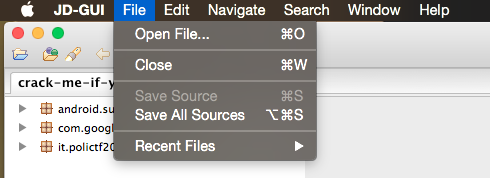# PoliCTF 2015 - Crack me if you can - Reversing 100

I untarred the challenge and in the folder: crack-me-if-you-can.apk. Awesome, I love reversing Android apps. I did what I always do when I get an apk: decompress and decompile it.

``````~/Dev/dex2jar/d2j-dex2jar.sh crack-me-if-you-can.apk
apktool d crack-me-if-you-can.apk
``````

Apktool complained about some of the resources since I don't have a framework-res.apk handy, but that's alright, I didn't need it to fully decompress anyway. Next, I loaded the jar file into JD-GUI and saved all of the sources from it as shown below.Then I unzipped and inspected the LoginActivity.java source file to see what the program was doing.

``````mkdir sources &amp;&amp; unzip sources.zip -d \$\_
``````

When reversing an android app I always start at the first activity and look at the OnCreate function.

``````protected void onCreate(Bundle paramBundle)
{
super.onCreate(paramBundle);
setContentView(2130968599);
if ((a(getApplicationContext(), 2)) \|\| (a(getApplicationContext(), "flagging{It_cannot_be_easier_than_this}")) \|\| (a(getApplicationContext(), false)) \|\| (a(getApplicationContext(), 2.78D)))
Toast.makeText(getApplicationContext(), getString(2131492925), 1).show();
while (true)
{
this.a = ((EditText)findViewById(2131361877));
((Button)findViewById(2131361878)).setOnClickListener(new a(this));
this.b = findViewById(2131361875);
return;
Toast.makeText(getApplicationContext(), getString(2131492922), 1).show();
}
}
``````

It is doing a check for a couple of different things and then showing a toast message. To figure out what that toast message is we can use aapt and the decompressed res files from apktool:

``````aapt d resources crack-me-if-you-can.apk | grep -i \$(printf "0x%x" 2131492925)
spec resource 0x7f0c003d it.polictf2015:string/ù: flags=0x00000000
resource 0x7f0c003d it.polictf2015:string/ù: t=0x03 d=0x00000180 (s=0x0008 r=0x00)
grep 'ù' crack-me-if-you-can/res/values/strings.xml
"Nice emulator, I'm watching you ;)"
Hello!
``````

It looks like that condition is just an emulator check, inspecting the functions closer confirms this (I don't need to show that here). Then an EditText area is created along with a button that has an onclick listener of a(this).

``````private void a()
{
this.a.setError(null);
String str = this.a.getText().toString();
boolean bool = TextUtils.isEmpty(str);
EditText localEditText = null;
int i = 0;
if (bool)
{
this.a.setError(getString(2131492923));
localEditText = this.a;
i = 1;
}
if ((!TextUtils.isEmpty(str)) &amp;&amp; (!a(str)))
{
this.a.setError(getString(2131492919));
localEditText = this.a;
i = 1;
}
if (i != 0)
localEditText.requestFocus();
}
``````

Everything in this is fairly straightforward: clear the error, get the string from the EditText area, make sure the string is not empty, if a(str) is false set an error, then focus on the text box again if there was an error. The function a(str) is the only thing interesting here.

``````private boolean a(String paramString)
{
if (paramString.equals(c.a(b.a(b.b(b.c(b.d(b.g(b.h(b.e(b.f(b.i(c.c(c.b(c.d(getString(2131492920))))))))))))))))
{
Toast.makeText(getApplicationContext(), getString(2131492924), 1).show();
return true;
}
return false;
}
``````

We want this function to return true so we need the paramString (the user input) to equal whatever that long mess of functions returns. To confirm that this is where we want to be looking, I found the string resource for the toast.

``````aapt d resources crack-me-if-you-can.apk  grep -i \$(printf "0x%x" 2131492924)
spec resource 0x7f0c003c it.polictf2015:string/ìò: flags=0x00000000
resource 0x7f0c003c it.polictf2015:string/ìò: t=0x03 d=0x0000017f (s=0x0008 r=0x00)
grep 'ìò' crack-me-if-you-can/res/values/strings.xml
Good to go! =)
``````

It looks like this is what we want. Now for the string in the mass on functions.

``````aapt d resources crack-me-if-you-can.apk | grep -i \$(printf "0x%x" 2131492920)
spec resource 0x7f0c0038 it.polictf2015:string/àè: flags=0x00000000
resource 0x7f0c0038 it.polictf2015:string/àè: t=0x03 d=0x0000017b (s=0x0008 r=0x00)
grep 'àè' crack-me-if-you-can/res/values/strings.xml
[[c%l][c{g}[%{%Mc%spdgj=]T%aat%=O%bRu%sc]c%ti[o%n=Wcs%=No[t=T][hct%=buga[d=As%=W]e=T%ho[u%[%g]h%t[%}%
``````

Now, the only thing left to do is look at the c and b classes (included with the challenge).

``````public class c
{
public static String a(String paramString)
{
return paramString.replace("aa", "ca");
}
public static String b(String paramString)
{
return paramString.replace("aat", "his");
}
public static String c(String paramString)
{
return paramString.replace("buga", "Goo");
}
public static String d(String paramString)
{
return paramString.replace("spdgj", "yb%e");
}
}
``````

b is basically the same thing, but obviously different substitutions and some replaceFirst functions as well. Now all that I needed to do was make the correct replacements on the string and the flag would reveal itself! I've never been good at java, so I just copied and pasted to python to solve.

``````c.a(b.a(b.b(b.c(b.d(b.g(b.h(b.e(b.f(b.i(c.c(c.b(c.d(getString(2131492920))
paramString = "[[c%l][c{g}[%{%Mc%spdgj=]T%aat%=O%bRu%sc]c%ti[o%n=Wcs%=No[t=T][hct%=buga[d=As%=W]e=T%ho[u%[%g]h%t[%}%"
paramString = paramString.replace("spdgj", "yb%e") #c.d
paramString = paramString.replace("aat", "his") #c.b
paramString = paramString.replace("buga", "Goo") #c.c
paramString = paramString.replace("=", "\_") #b.i
paramString = paramString.replace("\\}", "", 1) #b.f
paramString = paramString.replace("\\{", "", 1) #b.e
paramString = paramString.replace("R", "f", 1) #b.h
paramString = paramString.replace("c", "f", 1) #b.g
paramString = paramString.replace("]", "") #b.d
paramString = paramString.replace("[", "") #b.c
paramString = paramString.replace("%", "") #b.b
paramString = paramString.replace("c", "a") #b.a
paramString = paramString.replace("aa", "ca") #c.a
print(paramString)
``````

And then to run

``````python sol.py
fla{g}{Maybe_This_Obfuscation_Was_Not_That_Good_As_We_Thought}
``````

The flag was actually flag{Maybe_This_Obfuscation_Was_Not_That_Good_As_We_Thought} Some jerk posted this flag in IRC at like 2 in the morning. That was sort of dumb. Although only just over half of the teams got it.